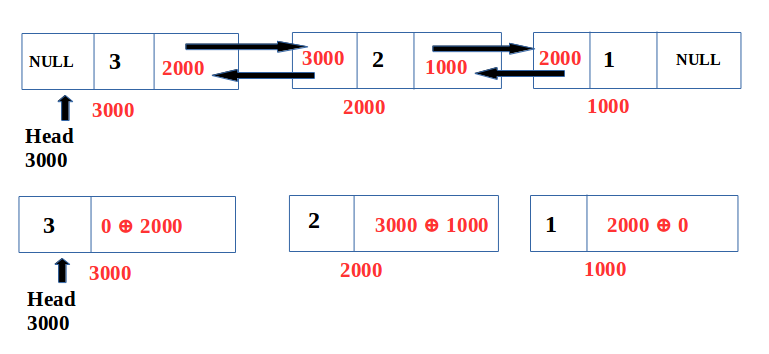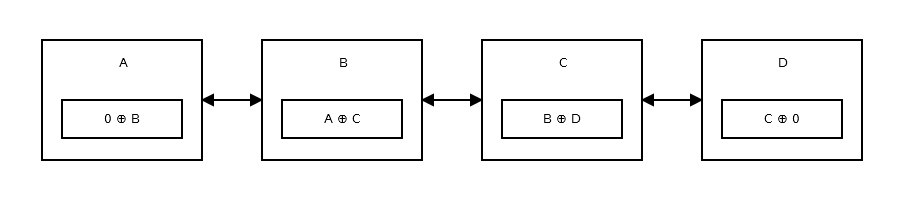×

Search anything:Get this book -> Problems on Array: For Interviews and Competitive Programming

Reading time: 35 minutes | Coding time: 12 minutes

XOR Linked List is the memory efficient version of Doubly Linked List because it makes use of only one space for address field with every node.
An ordinary doubly linked list stores addresses of the previous and next list items in each list node, requiring two address fields to be maintained per node:

• prev
• next

Whereas in XOR linked list, only one address field is maintained whose value is determined by the XOR of the previous node address and the next node address:

We will look into how XOR Linked List is useful and how it makes use of XOR operation. Further, the advantages and disadvantages will be discussed

Let us first look into the properties of XOR operation:

• X ⊕ X = 0
• X ⊕ 0 = X
• X ⊕ Y = Y⊕X
• (X ⊕ Y) ⊕ Z = X ⊕ ( Y ⊕ Z)

Just like Doubly Linked List, XOR Linked List also provides facilitates list traversal in both forward and backward directions. While traversing the list we need to remember the address of the previously accessed node in order to calculate the next node’s address.

The following terminologies will be used for describing the various sections of a node in Doubly linked list :

• data : The data is stored here
• link : The address of the next node is saved here
• head : points to the first node of the list
• tail : points to the last node of the listConsidering the properties of XOR operations as discussed above , we get the following results:

Bitwise XOR of NULL with address of node B

-> 0 ⊕ 2000 = 2000 ->Address of B

Bitwise XOR of NULL with address of node B

-> 3000 ⊕ 1000

Bitwise XOR of NULL with address of node B

-> 2000 ⊕ 0 = 2000 ->Address of B

### Traversing

We need to maintain the address of the previous node in some temporary pointer. By XORing the value in the link field of the current node with the address of the previous node, we can get the address of the next nodeA) From left to right :

i)For example, if we are node B and we want to move to node C.

ii)Suppose we are the initial node , then to go to the next node
-> NULL ⊕ (NULL ⊕ addr(B))

B) Right to left :
i)Lets start from the last node D and move to node C
-> NULL ⊕ (addr(C) ⊕ NULL)

ii) Now for the case where our current node is somewhere in the middle of the list , consider our current node is C, we can get the address of previous node B as

### Basic Operations

Following are the basic operations that are done on any linked list :

• Insertion
• Deletion

### Insertion

For implementation, the code given below will insert at the begining or at the end depending on the value of the second parameter 'at_tail' which is set to true if insertion has to take place at the end of the list otherwise it is set to false.

Pseudocode:

``````    step-1 : if list is empty
insert at the begining
goto step-4
step-2 : if at_tail is true
insert at the end
goto step-4
step-3 : else insert at begining
step-4 : end
``````

### Deletion

Deletion also takes place based on the 'at_tail' boolean parameter.If its value is true, then the last node will be deleted else the first node will be deleted.

Pseudocode:

``````    step-1 : if list is empty
display appropriate message that list is empty
goto step-5
step 2 : Store the value of the node in a variable 'item'
step-3 : Update the link field of the previous node if at_tail is true else                  update the link field of the next node i.e., deletion is taking place                at begining
step-5 : return the variable item
step-6 : end
``````

### Implementations

``````#include <stdio.h>
#include <stdlib.h>
#include <stdint.h>
#include <stdbool.h>

typedef struct node {
int item;
} node;

//Performs the xor operation of two pointers
node *xor(node *a, node *b)
{
return (node*) ((uintptr_t) a ^ (uintptr_t) b);
}

//insertion takes place based on the 'at_tail' boolean value
void insert(int item, bool at_tail)
{
node *ptr = (node*) malloc(sizeof(node));
ptr->item = item;

} else if (at_tail) {
tail = ptr;
} else {
}
}
//deletion takes place from the end
int delete(bool from_tail)
{
int item;
node *ptr;
printf("Empty list.\n");
exit(1);
} else if (from_tail) {
ptr = tail;
item = ptr->item;
if (NULL == prev) head = NULL;
tail = prev;

} else {
item = ptr->item;
if (NULL == next) tail = NULL;
}
free(ptr);
ptr = NULL;
return item;
}

void display()
{
node *prev = NULL, *next;

printf("\nList elements are : ");
while (NULL != curr) {
printf("%d ", curr->item);
prev = curr;
curr = next;
}

printf("\n");
}

int main(int argc, char *argv[])
{
int item;
for (int i = 1; i <= 10; i++){
insert(i, i < 6);
printf("Successfully inserted %d\n",i);
}

display();
printf("\n");
for (int i = 1; i <= 4; i++){
item=delete(i < 3);
printf("Successfully deleted %d\n",item);
}

display();
}
``````

### Complexity

These results are based on the above implementation :

1. Insertion
• Worst case time complexity: `Θ(1)`
• Average case time complexity: `Θ(1)`
• Best case time complexity: `Θ(1)`
• Space complexity: `Θ(1)`
2. Deletion
• Worst case time complexity: `Θ(1)`
• Average case time complexity: `Θ(1)`
• Best case time complexity: `Θ(1)`
• Space complexity: `Θ(1)`
3. Display
• Worst case time complexity: `Θ(n)`
• Average case time complexity: `Θ(n)`
• Best case time complexity: `Θ(n)`
• Space complexity: `Θ(1)`

### Applications

XOR/Memory Efficient linked list have same functionality as double linked list, they just need one one cell for both next and previous node link, so what ever real life usage of double linked list also applied to XORed linked list too. Some of them are listed below :

• Used for implementing the backward and forward navigation of visited web pages i.e. back and forward button.
• Used in applications that have a Most Recently Used list (a linked list of file names)

• Reduces memory usage
• Another advantage which might be useful is the ease of reversability.
• Reversing a doubly linked list invloves updating of all the prev and next values in all nodes. But we don’t have these pointers anymore as they are XOR-ed into one value. That means that we do not have to change anything, only the head pointer need to be changed to point to last node!